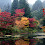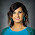## 7.13.2010

### Algebra 1 CRS Skills

I'm in Illinois and we are assessed by the ACT.

I went through the ACT College Readiness Standards and pulled out what I think is doable in Algebra 1.

...

Perform one-operation computation with whole numbers and decimals.

Solve problems in one or two steps using whole numbers.

Perform common conversions

Calculate the average of a list of positive whole numbers.

Perform a single computation using information from a table or chart.

Recognize equivalent fractions and fractions in lowest terms.

Exhibit knowledge of basic expressions (e.g., identify an expression for a total as b + g)

Solve equations in the form x + a = b, where a and b are whole numbers or decimals.

Identify the location of a point with a positive coordinate on the number line.

Locate points on the number line and in the first quadrant.

Solve routine one-step arithmetic problems (using whole numbers, fractions, and decimals) such as single-step percent.

Solve some routine two-step arithmetic problems.

Recognize one-digit factors of a number.

Identify a digit's place value.

Calculate the average of a list of numbers.

Perform computations on data from tables and graphs.

Substitute whole numbers for unknown quantities to evaluate expressions.

Solve one-step equations having integer or decimal answers.

Combine like terms (e.g., 2x + 5x).

Compute the perimeter of polygons when all side lengths are given.

Compute the area of rectangles when whole number dimensions are given.

Compute the area and perimeter of triangles and rectangles in simple problems.

Solve routine two-step or three-step arithmetic problems involving concepts such as rate and proportion, tax added, percentage off, and computing with a given average.

Translate from one representation of data to another (e.g., a bar graph to a circle graph).

Exhibit knowledge of elementary number concepts including rounding, the ordering of decimals, pattern identification, absolute value, primes, and greatest common factor.

Evaluate algebraic expressions by substituting integers for unknown quantities.

Add and subtract simple algebraic expressions.

Solve routine first-degree equations.

Perform straightforward word-to-symbol translations.

Multiply two binomials*.

Comprehend the concept of length on the number line*.

Solve multistep arithmetic problems that involve planning or converting units of measure (e.g., feet per second to miles per hour).

Manipulate data from tables and graphs.

Find and use the least common multiple.

Order fractions.

Work with numerical factors.

Work with scientific notation.

Work with squares and square roots of numbers.

Work problems involving positive integer exponents*.

Solve real-world problems using first-degree equations.

Write expressions, equations, or inequalities with a single variable for common pre-algebra settings (e.g., rate and distance problems and problems that can be solved by using proportions).

Identify solutions to simple quadratic equations.

Factor simple quadratics (e.g., the difference of squares and perfect square trinomials)*.

Solve first-degree inequalities that do not require reversing the inequality sign*.

Identify the graph of a linear inequality on the number line.*

Determine the slope of a line from points or equations*.

Match linear graphs with their equations*.

Solve word problems containing several rates, proportions, or percentages.

Apply number properties involving positive/negative numbers.

Apply rules of exponents.

Manipulate expressions and equations.

Write expressions, equations, and inequalities for common algebra settings.

Solve linear inequalities that require reversing the inequality sign.

Solve absolute value equations.

Find solutions to systems of linear equations.

Match number line graphs with solution sets of linear inequalities.

Use the Pythagorean Theorem.

Use the distance formula.

1.Why this random order? What's the normal teaching progression?
Also from my point of view the levels required to acquire these abilities are really different: it seems to me that those are abilities from elementary level to a 9 or 10 grade, do you really teach all these things in an year only?
What do students know usually by the end of middle school?

2.Students are expected to have a basic understanding of quite a few of these topics -- however many of them come to high school without these understandings in place. This is why Algebra 1 has become the problematic class for students to pass in order to graduate on time. There is so much to cover and "master" that when a group of students come to Alg 1 without knowing their multiplication facts or how to deal with integers it becomes almost futile to think that they can become proficient at all of these skills. Once a student has been introduced to these topics they become much easier in Algebra 2, but that won't usually be for another two years...

3.Ok, this list is very long and a bit overwhelming. I have a couple of suggestions.

I see that you wrote every standard from the CRS 13-27. I think your Algebra 1 kids are similar to my prep-level kids. Here's what I'd do:

Make a 3-column document in word. Label them "Already seen, need to review", "Learning focus", and "Extension-expose but don't expect mastery".

List everything 13-15 and maybe even some of the 16-19 in column 1. List the 16-19 & some of the 20-23 you feel are appropriate in column 2. THESE are your focus. It’s a shorter, more manageable list. List the rest of 20-23 and some of the 24-27 in column 3.

Ok, now for application. Take column 1 (your review) and make quick warm-up problems. Give these throughout the year to constantly spiral and support new learning. Take column 2 and find sections in the book that match up. This is what will be the bulk of what you teach, in depth. Take column 3 and find just a couple of problems for each standard. Expose the kids to them - some kids will get them and others will have no clue. But that's ok.

I hope this made sense...let me know if I can help in any way.

PS- I have materials on a lot of these standards. Let me know if you ever want to see stuff.

4.Silvia,
I pulled these from the ACT website and they are separated by strands and ACT score levels, 13-15, 16-19, etc. I just haven't put them in any order.

driesenberg,
Agreed.

Mr. D,
I'm using a college entrance exam because that's what Illinois uses as a state test. I'm trying to figure out which standards apply to what class. But you are right, I definitely have to teach them to decipher the skill from the given problem, which thanks to ACT, is no easy feat.

iTeach,
That makes perfect sense to me. Thanks again.